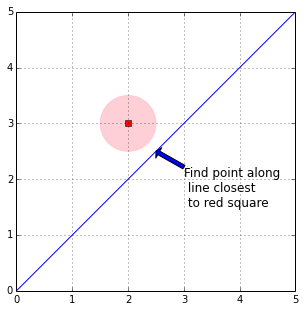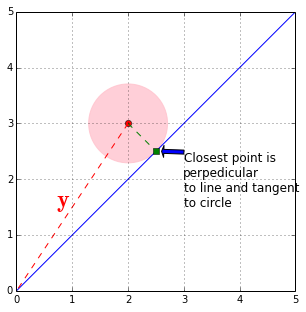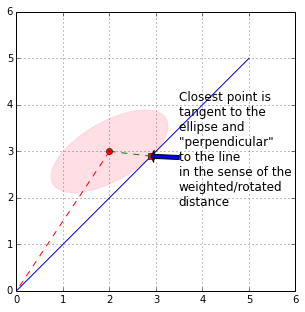On the road to a geometric understanding of conditional expectation, we need to grasp the concept of projection. In the figure below, we want to find a point along the blue line that is closest to the red square. In other words, we want to inflate the pink circle until it just touches the blue line. Then, that point will be the closest point on the blue line to the red square.

In :
from __future__ import division
from matplotlib import  patches

fig, ax = subplots()
fig.set_figheight(5)
x = arange(6)
y= matrix([,
])
ax.plot(x,x)
ax.plot(*y,marker='s',color='r')
ax.add_patch(patches.Circle(y,radius=.5,alpha=0.75,color='pink'))
ax.annotate('Find point along\n line closest\n to red square',
fontsize=12,xy=(2.5,2.5),
xytext=(3,1.5),
arrowprops={'facecolor':'blue'})
ax.axis(xmax=5,ymax=5)
ax.set_aspect(1)
ax.grid()It may be geometrically obvious, but the closest point on the line occurs where the line segment from the red square to the blue line is perpedicular to the line. At this point, the pink circle just touches the blue line. This is illustrated below.

In :
fig, ax = subplots()
fig.set_figheight(5)

x = arange(6)
y= matrix([,
])
ax.plot(x,x)
ax.plot(*y,marker='o',color='r')
ax.add_patch(patches.Circle(y,radius=1/sqrt(2.),alpha=0.75,color='pink'))

v = matrix([,])
Pv = v*v.T/ (v.T*v) # projection operator
ax.plot(*(Pv*y),marker='s',color='g')
ax.add_line( Line2D( (y[0,0], 2.5), (y[1,0],2.5) ,color='g',linestyle='--'))
ax.add_line( Line2D( (y[0,0], 0), (y[1,0],0) ,color='r',linestyle='--'))
ax.annotate( 'Closest point is\nperpedicular\nto line and tangent\nto circle',
fontsize=12,xy=(2.6,2.5),
xytext=(3,1.5),
arrowprops={'facecolor':'blue'})

ax.text(.7,1.5,r'$\mathbf{y}$',fontsize=24,color='r')
ax.set_aspect(1)
ax.grid()Now that we can see what's going on, we can construct the the solution analytically. We can represent an arbitrary point along the blue line as:

$$\mathbf{x} = \alpha \mathbf{v}$$

where $\alpha$ slides the point up and down the line with

$$\mathbf{v} = \left[ \begin{array}{c} 1 \\ 1 \\ \end{array} \right]$$

Formally, $\mathbf{v}$ is the subspace on which we want to project the $\mathbf{y}$. At the closest point, the vector between $\mathbf{y}$ and $\mathbf{x}$ (the dotted green error vector above) is perpedicular to the line. This means that

$$( \mathbf{y}-\mathbf{x} )^T \mathbf{v} = 0$$

and by substituting and working out the terms, we obtain

$$\alpha = \frac{\mathbf{y}^T\mathbf{v}}{|\mathbf{v}|^2}$$

The error is the distance between $\alpha\mathbf{v}$ and $\mathbf{y}$. Because we have a right triangle, using the Pythagorean theorem, we compute the squared length of this error as

$$\epsilon^2 = |( \mathbf{y}-\mathbf{x} )|^2 = |\mathbf{y}|^2 - \alpha^2 |\mathbf{v}|^2 = |\mathbf{y}|^2 - \frac{|\mathbf{y}^T\mathbf{v}|^2}{|\mathbf{v}|^2}$$

where $|\mathbf{v}|^2 = \mathbf{v}^T \mathbf{v}$. Note that since $\epsilon^2 \ge 0$, this also shows that

$$|\mathbf{y}^T\mathbf{v}| \le |\mathbf{y}| |\mathbf{v}|$$

which is the famous and useful Cauchy-Schwarz inequality which we will exploit later. Finally, we can assemble all of this into the projection operator

$$\mathbf{P}_v = \frac{1}{|\mathbf{v}|^2 } \mathbf{v v}^T$$

With this operator, we can take any $\mathbf{y}$ and find the closest point on $\mathbf{v}$ by doing

$$\mathbf{P}_v \mathbf{y} = \mathbf{v} \left( \frac{ \mathbf{v}^T \mathbf{y} }{|\mathbf{v}|^2} \right)$$

where we recognize the term in parenthesis as the $\alpha$ we computed earlier. It's called an operator because it takes a vector ($\mathbf{y}$) and produces another vector ($\alpha\mathbf{v}$).

### Weighted distances¶

We can easily extend this projection operator to cases where the measure of distance between $\mathbf{y}$ and the subspace $\mathbf{v}$ is weighted (i.e. non-uniform). We can accomodate these weighted distances by re-writing the projection operator as

$$\mathbf{P}_v = \mathbf{v}\frac{\mathbf{v}^T \mathbf{Q}^T}{ \mathbf{v}^T \mathbf{Q v} }$$

where $\mathbf{Q}$ is positive definite matrix. Earlier, we started with a point $\mathbf{y}$ and inflated a circle centered at $\mathbf{y}$ until it just touched the line defined by $\mathbf{v}$ and this point was closest point on the line to $\mathbf{y}$. The same thing happens in the general case with a weighted distance except now we inflate an ellipsoid, not a circle, until the ellipsoid touches the line.

The code and figure below illustrate what happens using the weighted $\mathbf{P}_v$. It is basically the same code we used earlier. You can download the IPython notebook corresponding to this post and try different values on the diagonal of $\mathbf{S}$ and $\theta$ below.

In :
theta = 120/180.*pi   # rotation angle for ellipse
v = matrix([,])

# rotation matrix
U = matrix([[ cos(theta), sin(theta)],
[-sin(theta), cos(theta)]])

# diagonal weight matrix
S = matrix([[5,0], # change diagonals to define axes of ellipse
[0,1]])
Q = U.T*S*U

Pv = (v)*v.T*(Q)/(v.T*(Q)*v)      # projection operator
err = sqrt((y-Pv*y).T*Q*(y-Pv*y)) # error length
xhat = Pv*y                       # closest point on line

fig, ax = subplots()
fig.set_figheight(5)

ax.plot(*y,marker='o',color='r')
ax.plot(x,x)
ax.plot(*(xhat),marker='s',color='r')
ax.add_patch( patches.Ellipse(y,err*2/sqrt(S[0,0]),err*2/sqrt(S[1,1]),
angle=theta/pi*180,color='pink',
alpha=0.5))
ax.add_line( Line2D( (y[0,0], 0), (y[1,0],0) ,color='r',linestyle='--'))
ax.add_line( Line2D( (y[0,0],xhat[0,0]),
(y[1,0],xhat[1,0]) ,
color='g',linestyle='--'))
ax.annotate( '''Closest point is
tangent to the
ellipse and
"perpendicular"
to the line
in the sense of the
weighted/rotated
distance
''',
fontsize=12,xy=(xhat[0,0],xhat[1,0]),
xytext=(3.5,1.5),
arrowprops={'facecolor':'blue'})

ax.axis(xmax=6,ymax=6)
ax.set_aspect(1)
ax.grid()Note that the error vector ( $\mathbf{y}-\alpha\mathbf{v}$ ) is still perpendicular to the line (subspace $\mathbf{v}$), but it doesn't look it because we are using a weighted distance. The difference between the first projection ( with the uniform circular distance) and the general case ( with the ellipsoidal weighted distance ) is the inner product between the two cases. For example, in the first case we have $\mathbf{y}^T \mathbf{v}$ and in the weighted case we have $\mathbf{y}^T \mathbf{Q}^T \mathbf{v}$. To move from the uniform circular case to the weighted ellipsoidal case, all we had to do was change all of the vector inner products. This is a conceptual move we'll soon use again.

Before we finished, we will need a formal property of projections:

$$\mathbf{P}_v \mathbf{P}_v = \mathbf{P}_v$$

known as the idempotent property which basically says that once we have projected onto a subspace, further subsequent projections leave us in the same subspace.

## Summary¶

In this section, we developed the concept of a projection operator, which ties a minimization problem (closest point to a line) to an algebraic concept (inner product). It turns out that these same geometric ideas from linear algebra can be translated to the conditional expectation. How this works is the subject of our next post.

This post was created using the nbconvert utility from the source IPython Notebook which is available for download from the main github site for this blog. The projection concept is masterfully discussed in the classic Strang, G. (2003). Introduction to linear algebra. Wellesley Cambridge Pr. Also, some of Dr. Strang's excellent lectures are available on MIT Courseware. I highly recommend these as well as the book.